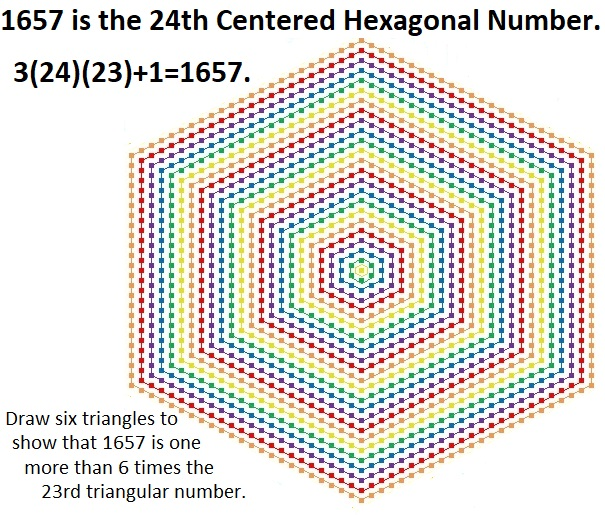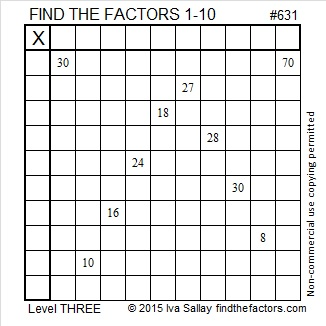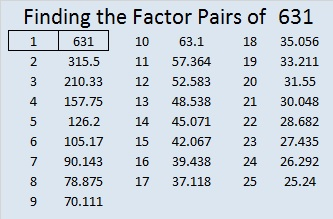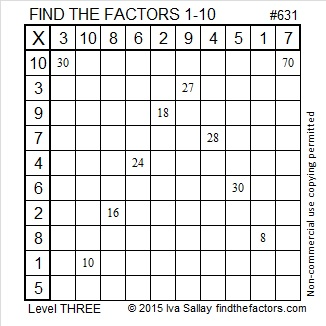# Prime Number 1657 is the 24th Centered Hexagonal Number

### Today’s Puzzle:

Draw six triangles on the graphic below to show that 1657 is one more than 6 times the 23rd triangular number.### Factors of 1657:

• 1657 is a prime number.
• Prime factorization: 1657 is prime.
• 1657 has no exponents greater than 1 in its prime factorization, so √1657 cannot be simplified.
• The exponent in the prime factorization is 1. Adding one to that exponent we get (1 + 1) = 2. Therefore 1657 has exactly 2 factors.
• The factors of 1657 are outlined with their factor pair partners in the graphic below.How do we know that 1657 is a prime number? If 1657 were not a prime number, then it would be divisible by at least one prime number less than or equal to √1657. Since 1657 cannot be divided evenly by 2, 3, 5, 7, 11, 13, 17, 19, 23, 29, 31, or 37, we know that 1657 is a prime number.

### More About the Number 1657:

1657 is the sum of two squares:
36² + 19² = 1657.

1657 is the hypotenuse of a primitive Pythagorean triple:
935-1368-1657, calculated from 36² – 19², 2(36)(19), 36² + 19².

Here’s another way we know that 1657 is a prime number: Since its last two digits divided by 4 leave a remainder of 1, and 36² + 19² = 1657 with 36 and 19 having no common prime factors, 1657 will be prime unless it is divisible by a prime number Pythagorean triple hypotenuse less than or equal to √1657. Since 1657 is not divisible by 5, 13, 17, 29, or 37, we know that 1657 is a prime number.

Do you notice anything else special about the number 1657 in this color-coded chart?# The Shape of 1387 Tiny Squares

22³ – 21³ = 1387, and that’s why it is the 22nd hexagonal number.1387 is also the 19th decagonal number. Why? Because 4(19²) – 3(19) = 1387.Here’s more about the number 1387:

• 1387 is a composite number.
• Prime factorization: 1387 = 19 × 73
• 1387 has no exponents greater than 1 in its prime factorization, so √1387 cannot be simplified.
• The exponents in the prime factorization are 1, and 1. Adding one to each exponent and multiplying we get (1 + 1)(1 + 1) = 2 × 2 = 4. Therefore 1387 has exactly 4 factors.
• The factors of 1387 are outlined with their factor pair partners in the graphic below.1387 is the hypotenuse of a Pythagorean triple:
912-1045-1387 which is 19 times (48-55-73)

# 1261 Can You Make a Star out of a Hexagon?

Can you make a star out of a hexagon? If you have 1261 tiny squares arranged as a centered hexagon, you can rearrange those 1261 tiny squares into a six-pointed star as illustrated below!37 was the last centered hexagonal number that was also a star number.

Here are some more facts about the number 1261:

• 1261 is a composite number.
• Prime factorization: 1261 = 13 × 97
• The exponents in the prime factorization are 1 and 1. Adding one to each and multiplying we get (1 + 1)(1 + 1) = 2 × 2 = 4. Therefore 1261 has exactly 4 factors.
• Factors of 1261: 1, 13, 97, 1261
• Factor pairs: 1261 = 1 × 1261 or 13 × 97
• 1261 has no square factors that allow its square root to be simplified. √1261 ≈ 35.510561261 is the sum of two squares two different ways:
30² + 19² = 1261
35² + 6² = 1261

1261 is the hypotenuse of FOUR Pythagorean triples:
420-1189-1261 calculated from 2(35)(6), 35² – 6², 35² + 6²
485-1164-1261 which is (5-12-13) times 97
539-1140-1261 calculated from 30² – 19², 2(30)(19), 30² + 19²
845-936-1261 which is 13 times (65-72-97)

# 1027 Find the Factors Challenge

I’m really enjoying these Find the Factors Challenge puzzles, and I hope that you will give them a try and love them, too. You can find this one as well as a little less challenging one in the excel file link below the puzzle. You can type the factors directly on that file. Remember to use logic for every single factor pair you use.Print the puzzles or type the solution in this excel file: 10-factors-1019-1027

Now I’ll tell you a little about the number 1027:

It is the sum of seven consecutive prime numbers:
131 + 137 + 139 + 149 + 151 + 157 + 163 = 1027

It is the sum of the squares of the first eight prime numbers:
2² +  3² +  5² +  7² +  11² +  13² +  17² +  19² = 1027
Indeed, 666 + 19² = 1027. Thanks to OEIS.org for that fun fact.

Because 19³ – 18³ = 1027, it is the 19th Centered Hexagonal Number.
That also means that 19² + 19(18) + 18² = 1027
because a³ – b³ = (a-b)(a²+ab+b²).1027 is the hypotenuse of a Pythagorean triple:
395-948-1027 which is (5-12-13) times 79.

1027 is also a palindrome when it is written in these three other bases:
717 in BASE 12
535 in BASE 14
1B1 in BASE 27 (B is 11 base 10)

• 1027 is a composite number.
• Prime factorization: 1027 = 13 × 79
• The exponents in the prime factorization are 1 and 1. Adding one to each and multiplying we get (1 + 1)(1 + 1) = 2 × 2 = 4. Therefore 1027 has exactly 4 factors.
• Factors of 1027: 1, 13, 79, 1027
• Factor pairs: 1027 = 1 × 1027 or 13 × 79
• 1027 has no square factors that allow its square root to be simplified. √1027 ≈ 32.0468

# Why Prime Number 919 is the 18th Centered Hexagonal Number

919 is a prime number, but if you had 919 little squares, they could be formed into this fabulous shape:This hexagon is made from 18 concentric hexagons using the pattern yellow, green, blue, purple, red, and orange repeated. You can easily count that there are 3 × 6 hexagons. (Yes, that’s counting the yellow square in the center as a hexagon because 1 is the first centered hexagonal number.) Here’s why prime number 919 is a centered hexagonal number:

919 = 1 + 6 + 12 + 18 + 24 + 30 + 36 + 42 + 48 + 54 + 60 + 66 + 72 + 78 + 84 + 90 + 96 + 102, the number of squares contained in those concentric hexagons listed in order from smallest to largest . Thus,
919 = 1 + 6(1 + 2 + 3 + 4 +  5 + 6 + 7 + 8 + 9 + 10 + 11 + 12 + 13 + 14 + 15 + 16 + 17) = 1 + 6(153)

919 is a centered hexagonal number because 919 – 1 is 918. What was special about 918? Well, consecutive numbers, 17 and 18, are two of its factors. That made the 17th triangular number a factor of 918. Because 918 is 6 times a triangular number (153), the next number, 919, is a centered hexagonal number.

919 is the 18th centered hexagonal number because 817 (the 17th centered hexagonal number) plus 6(17) = 919.

919 is the 18th centered hexagonal number because 630 (the 18th hexagonal number) plus 17² = 919:919 is also the 18th centered hexagonal number because 18³ – 17³ = 919. Even though the difference of two cubes can always be factored, 919 is still a prime number because
18³ – 17³ = (18 – 17)(18² + (17)(18) + 17²) = (1)(919)

919 is not only the 18th centered hexagonal number, but it is a palindrome in base 10 and two other bases:

414 in BASE 15

171 in BASE 27

919 uses its same digits, 199, in BASE 26

• 919 is a prime number.
• Prime factorization: 919 is prime.
• The exponent of prime number 919 is 1. Adding 1 to that exponent we get (1 + 1) = 2. Therefore 919 has exactly 2 factors.
• Factors of 919: 1, 919
• Factor pairs: 919 = 1 × 919
• 919 has no square factors that allow its square root to be simplified. √919 ≈ 30.31501

How do we know that 919 is a prime number? If 919 were not a prime number, then it would be divisible by at least one prime number less than or equal to √919 ≈ 30.3. Since 919 cannot be divided evenly by 2, 3, 5, 7, 11, 13, 17, 19, 23, or 29, we know that 919 is a prime number.# 631 and Level 3

631 is a prime number that happens to also be the sum of the eleven prime numbers from 37 to 79.

Sometimes mathematicians talk about triangular numbers, centered triangular numbers, hexagonal numbers, and centered hexagonal numbers. Is there any relationship between those terms?

• The sum of any three consecutive triangular numbers is a centered triangular number.
• ALL hexagonal numbers are triangular numbers.
• Most centered hexagonal numbers are NOT centered triangular numbers.

In my last post, I pointed out that 630 is a triangular number and a hexagonal number.

Well, amazingly the next number, 631, is a centered triangular number AND a centered hexagonal number.

How often does one more than a triangular number equal a centered triangular number? Also how often does one more than a hexagonal number equal a centered hexagonal number?

Let’s compare a couple of lists of these types of numbers up to 631. Red means a number is in both lists. Blue means the number in the 1st list is one less than the number in the 2nd list.

• Triangular numbers (with hexagonal numbers in bold type):
• 1, 3, 6, 10, 15, 21, 28, 36, 45, 55, 66, 78, 91, 105, 120, 136, 153, 171, 190, 210, 231, 253, 276, 300, 325, 351, 378, 406, 435, 465, 496, 528, 561, 595, 630.
• Centered triangular numbers (with numbers that are also centered hexagonal numbers in bold type):
• 1, 4, 10, 19, 31, 46, 64, 85, 109, 136, 166, 199, 235, 274, 316, 361, 409, 460, 514, 571, 631.

1 is the only number in the lists that is a triangular, centered triangular, hexagonal, and centered hexagonal number all at the same time. (1 is also a square number, a pentagonal number, etc.)

(630, 631) is the first combination of numbers that has BOTH a triangular number immediately followed by a centered triangular number AND a hexagonal number immediately followed by a centered hexagonal number.Print the puzzles or type the solution on this excel file: 10 Factors 2015-09-28

—————————————————————————————————

• 631 is a prime number.
• Prime factorization: 631 is prime.
• The exponent of prime number 631 is 1. Adding 1 to that exponent we get (1 + 1) = 2. Therefore 631 has exactly 2 factors.
• Factors of 631: 1, 631
• Factor pairs: 631 = 1 x 631
• 631 has no square factors that allow its square root to be simplified. √631 ≈ 25.11971.How do we know that 631 is a prime number? If 631 were not a prime number, then it would be divisible by at least one prime number less than or equal to √631 ≈ 25.1. Since 631 cannot be divided evenly by 2, 3, 5, 7, 11, 13, 17, 19, or 23, we know that 631 is a prime number.

—————————————————————————————————

A Logical Approach to solve a FIND THE FACTORS puzzle: Find the column or row with two clues and find their common factor. (None of the factors are greater than 10.)  Write the corresponding factors in the factor column (1st column) and factor row (top row).  Because this is a level three puzzle, you have now written a factor at the top of the factor column. Continue to work from the top of the factor column to the bottom, finding factors and filling in the factor column and the factor row one cell at a time as you go.